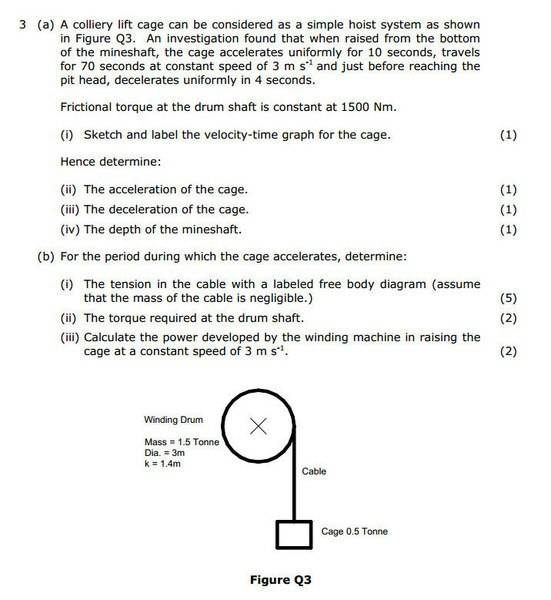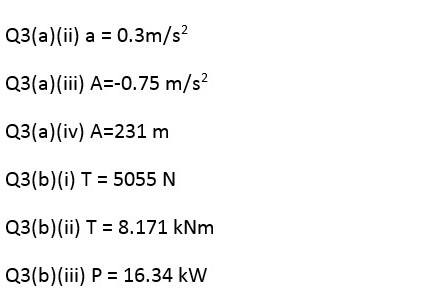# Torque Help -- Tension in a Hoist Cable

Hi, I'm having a problem with 3. (b) (ii) here.

I know that the frictional torque is 1500Nm and that from (b)(i) being 5055N I assume this is multiplied by 1.5M (drum shaft radius) which gives me 7582.5N. Do I have to calculate the angular acceleration of the drum from here, which could then give me the torque? And if so, how?

Help much appreciated. Answer and solution below.In addition to this how is the power calculated for (b)(iii)? I understand it is two times the torque but why?

Thanks.

CWatters
Homework Helper
Gold Member
Two times the torque?

Power = Torque * angular velocity

CWatters
Homework Helper
Gold Member
Hi, I'm having a problem with 3. (b) (ii) here.
I know that the frictional torque is 1500Nm and that from (b)(i) being 5055N I assume this is multiplied by 1.5M (drum shaft radius) which gives me 7582.5N. Do I have to calculate the angular acceleration of the drum from here, which could then give me the torque? And if so, how?

Yes.

First calculate the angular acceleration from the velocity of the rope and the dimensions of the drum.

Then what equation relates torque and angular velocity?

Last edited:
CWatters